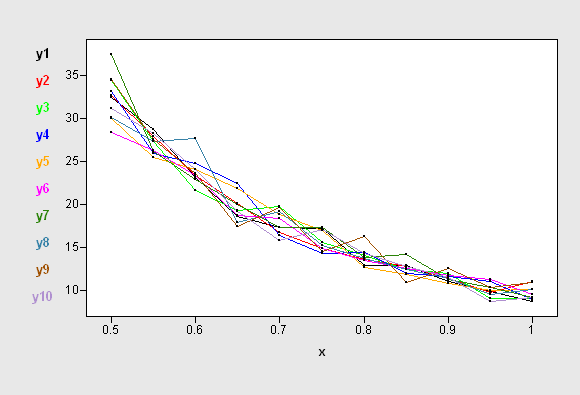## What does this message indicate?"WARNING: The point x is feasible only at the LCEPSILON= 10 range"

Dear all,

I ve asked  questions concerning this code before. I made some modifications to my code and i got the following message :"WARNING: The point x is feasible only at the LCEPSILON= 10 range". The progarm is supposed to do the following:

1- Min the integration of qq where the integration variable is "diff"

2-qq is a function of the vector hr of length 2x1

3-the vector hr is the vector of decesion variables which I am supposed to find using the NLPQN technique

4- the constraints in my model is formulated in the module qq0. The constraint is supposed to restrict bb0 to be =100 for the optimal values of hr and hr

I have three questions:

1-Is the qq0 module performing what intended?

2-The meaning of the warning message?

3- Your comment on the output.

THE LOG AND OUTPUT FILES ARE AS FOLLOWS:

1    proc iml;

2

3

4    *THE INTEGRAND MODULE;

5

6

7    start qq(diff)global(hr);

8    qqvector=0;

9    do i=1 to 100;

10   rl=0;

11   z=0;

12   do until(z>hr|z<-hr);

13   xbar=normal(-1)+diff;

14   z=hr*xbar+(1-hr)*z;

15   rl=rl+1;

16   end;

17   qqvector=qqvector//rl;

18   end;

19   bb=(sum(qqvector)/100);

20   return(bb);

21   finish;

NOTE: Module QQ defined.

22

23

24

25   *THE INTEGRATION OF qq WHICH IS MY OBJECTIVE FUNCTION;

26

27

28   start OBJ(hr);

29   a={0.5  1};

31   return(k);

32   finish OBJ;

NOTE: Module OBJ defined.

33

34

35   *THE CONSTraint MODULE;

36

37

38   start qq0(hr);

39   bb0=j(1,1,100.);

40   qqvector2=0;

41   do i=1 to 100;

42   rl2=0;

43   z2=0;

44   do until(z2>hr|z2<-hr);

45   xbar2=normal(-1);

46   z2=hr*xbar2+(1-hr)*z2;

47   rl2=rl2+1;

48   end;

49   qqvector2=qqvector2//rl2;

50   end;

51   bb0=sum(qqvector2)/100;

52   return(bb0);

53   finish qq0;

NOTE: Module QQ0 defined.

54

55

56    *THE NLP CALL;

57

58

59   hr={0.8 0.15};

60   optn= j(1,11,.);

60 !                  optn= 2;

60 !                             optn=1;

60 !                                        optn=1;

61   CALL NLPNMS(rc,hrres,"OBJ",hr,optn) nlc="qq0";

NOTE: ABSXCONV convergence criterion satisfied.

WARNING: The point x is feasible only at the LCEPSILON= 10 range.

62   quit;

NOTE: Exiting IML.

NOTE: 30389 workspace compresses.

NOTE: PROCEDURE IML used (Total process time):

THE OUTPUT FILE IS ATTACHED

Thanks,

Emara

4 REPLIES 4

## Re: What does this message indicate?"WARNING: The point x is feasible only at the LCEPSILON= 10 range"

I'm trying to understand what you are attempting, but it doesn't make sense to me.  Let's visualize what you are trying to do.

Compute 10 curves by calling your QQ function as follows. Each column of the Y matrix is a single instance of evaluating the QQ function on x={0.5, 0.55, 0.6, ..., 1}

x = T( do(0.5, 1, 0.05) );
y = j(nrow(x), 10);
do j = 1 to ncol(y);
do i = 1 to nrow(x);
y[i,j] = qq(x);
end;
end;

A graph of the 10 curves is shown below.Your objective function, OBJ, is the integral of the QQ function. Therefore, it seems to me that calling OBJ gives a random quantitiy.

This is assuming that the QUAD function converges, which it doesn't for me.  If I run

Integral = OBJ(hr); print Integral;

I get the error "Convergence could not be attained over the subinterval (0.5, 1)"

If any of my analysis is wrong, please clarify. As it is, I don't see how your program makes mathemtical sense. The NLP functions find the minimum of a deterministic function, not random quantities.

## Re: What does this message indicate?"WARNING: The point x is feasible only at the LCEPSILON= 10 range"

Dear Dr. Rick,

I dont understand exactly what is wrong, could u clarify more if possible. I will try to clarify more what I am doing. The idea is like  minimizing type two error(qq module in my syntax) ,fixing type one error (qq0 in my syntax) at a certain level for a hypothesis test in which the null hypothesis is that diff=0 versus the alternative hypothesis diff not=0 (diff is the integration variable in my syntax). However, in my program, I want to minimize "qq" over the whole range of the variable "diff" (0,0.5). That is why I am integrating qq over the variable diff as if I am obtaining an expected value of qq. I want to keep "type one error" at a certain level (100 in my syntax). Thus my objective function is "OBJ" and my non linear constraint is "qq0=100". Type one error and two errors in my syntax dont have a closed formula to be computed and are both computed using simulation (100 runs in my syntax). My aim is to find the values of hr and hr that gives me the minimum type two error , fixing type one error at  certain level (of course what is written in my syntax is not type one and two errors, but the idea is similar). Can't I do this using SAS? Please let me know if this is not possible using SAS or if I cant clarify what i am doing. I know that the minimization problem can be solved as it is done in several papers, but i want to solve it using SAS

Thank u for ur help,

Emara

## Re: What does this message indicate?"WARNING: The point x is feasible only at the LCEPSILON= 10 range"

Please list the papers that provide the reference for this method.

## Re: What does this message indicate?"WARNING: The point x is feasible only at the LCEPSILON= 10 range"

Here is the paper

"Optimization designs of the combined Shewhart-CUSUM control charts"